# Simulation Study of the Evolution of Cavity Erosion in High Voltage Electrical Cables

Simulation Study of the Evolution of Cavity Erosion in High Voltage Electrical Cables
National Polytechnic School / Electrical Engineering El Harrach Algiers-Algeria- 10 Avenue Hassen Badi BP 182 El Harrach 16200 Algiers, Algeria

Faculty of Technology, Department of Electrical Engineering, University Amar Telidji Road Ghardaia BP37G-Laghouat, Algeria

Corresponding Author Email:
allalnouar@gmail.com
Page:
98-109
|
DOI:
https://doi.org/10.18280/mmc_a.922-410
15 June 2019
|
Accepted:
17 August 2019
|
Published:
30 December 2019
| Citation

OPEN ACCESS

Abstract:

The reliability of these cables was satisfactory, but their implementation had major drawbacks unwinding and pulling difficulties (due to the rigidity and the mass of cable), the long and delicate junction. At present, the replacement of insulated cables with paper impregnated with synthetic insulated cables has been evoked for reasons of the excellent qualities of synthetic insulators. Electrical stresses are essential parameters in the breakage of insulating materials such that the thickness of the insulator, or the minimum distance required between a conductor and ground that will depend on this maximum stress value. The importance of the study of the electric field in high voltage is essential because it constitutes: the most important constraint. The calculation of the electric field is governed by the Poisson's equation or Laplace. The resolution of these equations requires the knowledge of certain conditions on the boundaries of the studied domain. There are two main families of solving methods, one is analytical, gives exact values, and the other is approximate and is based on numerical methods.

Keywords:

high voltage power cable, electrical trees, insulating materials, breakdown mechanisms, the finite difference method

1. Introduction

The distribution of the electric field, in most insulation systems, is determined by simulation using numerical analysis techniques. The approximation techniques used in numerical analysis are numerous and all provide a suitable calculation algorithm. The finite difference method is the simplest. It was the first to be used for the study of high voltage electric fields. It is a method of numerical resolution of partial differential equations, based on the approximation of the differential operator by a finite difference operator. In the finite difference method the partial differential equations are replaced by a set of finite difference equations expressed at the nodes of a distributed point network in the modeled domain. We obtain at the end a set of linear equations which, once solved, gives an approximate distribution of the sought variable. This is a simple method, which is very suitable for symmetrical or two-dimensional geometry systems. Indeed, the axial symmetry of the electric cable makes it possible to reduce the dimensions of the area of calculation of the electric field to the plane corresponding to the cross section of the cable. The choice of the polar coordinates r and q in this case is necessary and because of this, the electric field at any point of the insulation will have the value and the direction of the resultant of two components, one radial and the other tangential. [1-5].

2. Description of Analyzed

The numerical calculation of the electric field is done by solving the Poisson equation using the finite difference method. Generally, the density of free charges in dielectric materials is negligible. In this case, the problem becomes again a resolution of the Laplace equation. In a first approach, we will give the analytical expression of the distribution of the electric field in a perfect cable with homogeneous and isotropic insulation. The analytical model will be used as a reference to confirm the validity of the numerical method. [6-9].

## 1.jpgFigure 1. Presentation of a high voltage electrical cable

The potential equation obtained by applying the Gauss theorem is:

$V(r_{i})=\frac{V_{c}}{\ln \left(\frac{R}{r_{c}}\right)} \cdot \ln \left(\frac{R}{r_{i}}\right)$      (1)

ri: given position

With the following boundary conditions:

- Potential of the soul:

- Potential of the outer metallic sheath:

The expression of the field is obtained by derivation of V (ri) with respect to rI, $\vec{E}=-\operatorname{grad} \vec{V}$,

From where

$E\left(r_{i}\right)=\frac{V_{c}}{r_{i}} \cdot \ln \frac{R}{r_{c}}$      (2)

2.1 Mesh of a section of a cable

Figure 2 shows the mesh of a cross-section of a cable. We denote by hr the radial pitch and dq the angular pitch. We choose a sector defined by an angle a, such as:

$\alpha=\frac{2 \pi}{n}[r a d] ; d \theta=\frac{\alpha}{N-1}[r a d] ; h_{r}=\frac{R-r_{c}}{M-1}$      (3)

where R is the outer radius of the cable, rc is the radius of the conductive core, N and M are the number of radial and circular grid lines, n is the number defining the sector of the study area

## 2.jpgFigure 2. Division of the cable section into sectors

2.2 Case of a cable with homogeneous insulation

It is a question of finding the distribution of the potential in the insulation of the cable and of making a verification of the validity of the program by comparing its results with those of the analytical computation.

## 3.jpg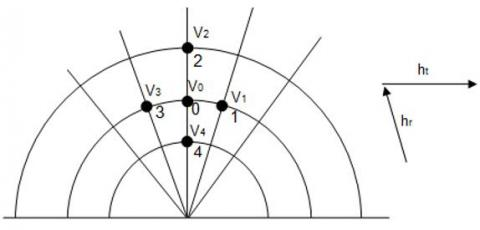Figure 3. Nodes from the discretization of the domain

The Laplace equation given in polar coordinates at the point O is written:

$\left.\frac{\partial^{2} V}{\partial r^{2}}\right|_{0}+\left.\frac{1}{r} \frac{\partial V}{\partial r}\right|_{0}+\left.\frac{1}{r^{2}} \frac{\partial^{2} V}{\partial \theta^{2}}\right|_{0}=0$      (4)

To replace the partial derivatives in this equation at the point considered, by the potential in this point, and the neighboring points, we proceed by Taylor series development of the function V(r, q) at points 1, 2, 3 and 4 , which gives the following system of equations:

$V_{1}=V_{0}+h_{t} \frac{\partial V}{\partial \theta}+\frac{h_{t}^{2}}{2} \cdot \frac{\partial^{2} V}{\partial \theta^{2}}$      (5)

$V_{2}=V_{0}+h_{r} \frac{\partial V}{\partial r}+\frac{h_{r}^{2}}{2} \cdot \frac{\partial^{2} V}{\partial r^{2}}$      (6)

$V_{3}=V_{0}-h_{t} \frac{\partial V}{\partial \theta}+\frac{h_{t}^{2}}{2} \cdot \frac{\partial^{2} V}{\partial \theta^{2}}$      (7)

$V_{4}=V_{0}-h_{r} \frac{\partial V}{\partial r}+\frac{h_{r}^{2}}{2} \cdot \frac{\partial^{2} V}{\partial r^{2}}$      (8)

By combining equations (5) and (6); (7) and (8), we obtain:

$\frac{\partial^{2} V}{\partial \theta^{2}}=\frac{V_{1}+V_{3}-2 V_{0}}{h_{t}^{2}}$      (9)

$\frac{\partial^{2} V}{\partial r^{2}}=\frac{V_{2}+V_{2}-2 V_{0}}{h_{r}^{2}}$      (10)

$\frac{\partial V}{\partial \theta}=\frac{V_{1}-V_{3}}{2 h_{t}}$      (11)

$\frac{\partial V}{\partial r}=\frac{V_{2}-V_{4}}{2 h_{r}}$      (12)

And by replacing the partial derivatives (9), (10), (11) and (12) in the Laplace equation, we will have:

$\frac{1}{h_{r}^{2}}\left(V_{2}+V_{4}-2 V_{0}\right)+\frac{1}{2 r h_{r}}\left(V_{2}-V_{4}\right)+\frac{1}{r^{2} h_{t}^{2}}\left(V_{1}+V_{3}-2 V_{0}\right)=0$      (13)

Then one can write the general potential equation in each node in the following form:

$V_{0}=\frac{r^{2} h_{t}^{2} h_{r}^{2}}{2\left(r^{2} h_{t}^{2}+h_{r}^{2}\right)}\left[\begin{array}{c}\frac{1}{r^{2} h_{t}^{2}} V_{1}+\frac{\left(2 r+h_{r}\right)}{2 r h_{r}^{2}} V_{2} \\ +\frac{1}{r^{2} h_{t}^{2}} V_{3}+\frac{2 r-h_{r}}{2 r h_{r}^{2}} V_{4}\end{array}\right]$      (14)

Written in the index form (i, j) we will have:

$V(i, j)=\frac{r_{i}^{2} h_{t}^{2} h_{r}^{2}}{2\left(r_{i}^{2} h_{t}^{2}+h_{r}^{2}\right)}\left[\begin{array}{l}\frac{1}{r_{i}^{2} h_{t}^{2}} V(i, j+1) \\ +\frac{2 r_{i}+h_{r}}{2 r_{r} h_{r}^{2}} V(i+1, j) \\ +\frac{1}{r_{i}^{2} h_{t}^{2}} V(i, j-1) \\ +\frac{2 r_{i}-h_{r}}{2 r_{i} h_{r}^{2}} V(i-1, j)\end{array}\right]$      (15)

2.3 Case of an insulated cable containing a cavity

In this part, we give the expressions of potential to the different nodes, including erosion. [10-14].

## 4.jpg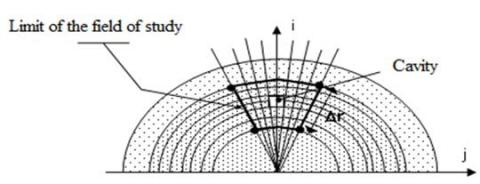Figure 4. Area of influence

- The permittivity insulation ei

- The cavity of permittivity ec

- The boundaries separating the two environments.

-rid: radial position of the center of the cavity relative to the center of the cable.

- $\Delta r$=(r2-r1): radial length of the domain

- $\alpha$: angular spacing defining the zone of influence.

The range of influence of the cavity therefore extends over a zone of radius approximately 10 times the radius of the cavity.

3. Approximation of Potential at Different Nodes

- For the nodes located in the insulator or inside the defect, the potential is given by the expression (15).

- For the nodes located at the border between the two mediums, one applies the conditions of continuity of the electric field considering what is known by the condition of Cauchy.

The shape of the cavity resulting from the discretization has a practically square geometry. The interface nodes between the cavity and the insulator will in this case be rectilinear and rectangular types,be:

## 5.jpgFigure 5. Mesh of the cavity

The continuity conditions at the interface between the insulator and the cavity are expressed by the following two equalities:

$\varepsilon_{i}\left(\frac{\partial V}{\partial r}\right)=\varepsilon_{c}\left(\frac{\partial V}{\partial r}\right)$

$\varepsilon_{i}\left(\frac{\partial V}{\partial \theta}\right)=\varepsilon_{c}\left(\frac{\partial V}{\partial \theta}\right)$      (16)

Depending on whether the electric field vector crosses the node in the radial or transverse direction.

3.1 Nodes of straight borders

a) -Node located at the top

$\left.V_{1}=V_{0}+h_{t} \frac{\partial V}{\partial \theta}+\frac{h_{t}^{2}}{2} \frac{\partial^{2} V}{\partial \theta^{2}}\right\} \cdot \frac{1}{r^{2} h_{t}^{2}}$      (17)

$\left.V_{2}=V_{0}+h_{r} \frac{\partial V}{\partial r}+\frac{h_{r}^{2}}{2} \frac{\partial^{2} V}{\partial r^{2}}\right\} \cdot \frac{1}{h_{r}^{2}}$      (18)

$\left.V_{3}=V_{0}-h_{t} \frac{\partial V}{\partial \theta}+\frac{h_{t}^{2}}{2} \frac{\partial^{2} V}{\partial \theta^{2}}\right\} \cdot \frac{1}{r^{2} h_{t}^{2}}$      (19)

$\left.V_{4}=V_{0}-h_{r} \frac{\partial V}{\partial r}+\frac{h_{r}^{2}}{2} \frac{\partial^{2} V}{\partial r^{2}}\right\} \cdot \frac{1}{h_{r}^{2}}$      (20)

Adding Eqns. (17), (18) and (19), we find:

$\frac{V_{1}}{r^{2} h_{t}^{2}}+\frac{2 V_{2}}{h_{r}^{2}}+\frac{V_{3}}{r^{2} h_{t}^{2}}=V_{0}\left(\frac{2}{r^{2} h_{t}^{2}}+\frac{2}{h_{r}^{2}}\right)+\frac{2}{h_{r}} \cdot \frac{\partial V}{\partial r}+\frac{\partial^{2} V}{\partial r^{2}}+\frac{1}{r^{2}} \cdot \frac{\partial^{2} V}{\partial \theta^{2}}$      (21)

From where we draw the expression $\left(\frac{\partial V}{\partial r}\right)_{I}$:

$\left(\frac{\partial V}{\partial r}\right)_{I}=\frac{r h_{r}}{2 r-h_{r}}\left[\begin{array}{l}\frac{V_{1}}{r^{2} h_{t}^{2}}+\frac{2 V_{2}}{h_{r}^{2}}+ \\ \left.\frac{V_{3}}{r^{2} h_{t}^{2}}-\frac{2\left(r^{2} h_{t}^{2}+h_{r}^{2}\right)}{r^{2} h_{t}^{2} h_{r}^{2}} \cdot V_{0}\right]\end{array}\right]$      (22)

Adding Equations (II.17) and (II.20), we find the expression of $\left(\frac{\partial V}{\partial r}\right)_{I I}$ is:

$\left(\frac{\partial V}{\partial r}\right)_{I I}=-\frac{r h_{r}}{2 r+h_{r}}\left[\begin{array}{l}\frac{V_{1}}{r^{2} h_{t}^{2}}+\frac{2 V_{4}}{h_{r}^{2}}+ \\ \frac{V_{3}}{r^{2} h_{t}^{2}}-\frac{2\left(r^{2} h_{t}^{2}+h_{r}^{2}\right)}{r^{2} h_{t}^{2} h_{r}^{2}} V_{0}\end{array}\right]$      (23)

According to Eqns. (18) and (19), one can write the continuity equation as follows:

$\varepsilon_{1}\left(\frac{\partial V}{\partial r}\right)_{I}=\varepsilon_{2}\left(\frac{\partial V}{\partial r}\right)_{I I}$

From which one derives the expression of V0:

$V_{0}=\frac{r^{2} h_{t}^{2} h_{r}^{2}}{2\left(r^{2} h_{t}^{2}+h_{r}^{2}\right)}\left[\begin{array}{l}\frac{1}{r^{2} h_{t}^{2}} V_{1}+\frac{2 \varepsilon_{1}\left(2 r+h_{r}\right)}{\left[\varepsilon_{1}\left(2 r+h_{r}\right)+\varepsilon_{2}\left(2 r-h_{r}\right)\right] h_{r}^{2}} V_{2} \\ +\frac{1}{r^{2} h_{t}^{2}} V_{3} \\ +\frac{2 \varepsilon_{2}\left(2 r-h_{r}\right)}{\left[\varepsilon_{1}\left(2 r+h_{r}\right)+\varepsilon_{2}\left(2 r-h_{r}\right)\right] h_{r}^{2}} V_{4}\end{array}\right]$      (24)

Thus, the general expression of the potential of the rectilinear nodes situated at the top will be given in the following index form:

$V(i, j)=\frac{r_{i}^{2} h_{t}^{2} h_{r}^{2}}{2\left(r_{i}^{2} h_{t}^{2}+h_{r}^{2}\right)}\left[\begin{array}{l}\frac{1}{r_{i}^{2} h_{t}^{2}} V(i, j+1) \\ +\frac{2 \varepsilon_{1}\left(2 r_{i}+h_{r}\right)}{\left[\varepsilon_{1}\left(2 r_{i}+h_{r}\right)+\varepsilon_{2}\left(2 r_{i}-h_{r}\right)\right] h_{r}^{2}} V(i+1, j) \\ +\frac{1}{r_{i}^{2} h_{t}^{2}} V(i, j-1) \\ +\frac{2 \varepsilon_{2}\left(2 r_{i}-h_{r}\right)}{\left[\varepsilon_{1}\left(2 r_{i}+h_{r}\right)+\varepsilon_{2}\left(2 r_{i}-h_{r}\right)\right] h_{r}^{2}} V(i-1, j)\end{array}\right]$      (25)

b) -Node located at the bottom

## 1.jpgThe expression of the potential is the same as the expression of the potential of the rectilinear nodes situated at the top only, one must switch between the permittivity e1 and e2, that is:

$V(i, j)=\frac{r_{i}^{2} h_{t}^{2} h_{r}^{2}}{2\left(r_{i}^{2} h_{t}^{2}+h_{r}^{2}\right)}\left[\begin{array}{c}\frac{1}{r_{i}^{2} h_{t}^{2}} V(i, j+1)+\frac{2 \varepsilon_{2}\left(2 r_{i}+h_{r}\right)}{\left[\varepsilon_{2}\left(2 r_{i}+h_{r}\right)+\varepsilon_{1}\left(2 r_{i}-h_{r}\right)\right] h_{r}^{2}} V(i+1, j) \\ +\frac{1}{r_{i}^{2} h_{t}^{2}} V(i, j-1)+\frac{2 \varepsilon_{1}\left(2 r_{i}-h_{r}\right)}{\left[\varepsilon_{2}\left(2 r_{i}+h_{r}\right)+\varepsilon_{1}\left(2 r_{i}-h_{r}\right)\right] h_{r}^{2}} V(i-1, j)\end{array}\right]$      (26)

c) -Node located on the right

## 2.jpg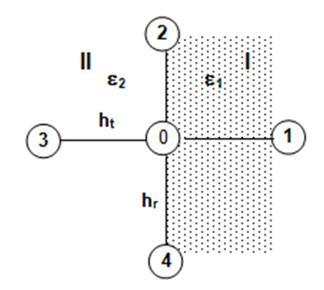$\left.V_{1}=V_{0}+h_{t} \frac{\partial V}{\partial \theta}+\frac{h_{t}^{2}}{2} \frac{\partial^{2} V}{\partial \theta^{2}}\right\} \cdot \frac{2}{r^{2} h_{t}^{2}}$      (27)

$\left.V_{2}=V_{0}+h_{r} \frac{\partial V}{\partial r}+\frac{h_{r}^{2}}{2} \frac{\partial^{2} V}{\partial r^{2}}\right\} \cdot \frac{1}{h_{r}^{2}}$      (28)

$\left.V_{3}=V_{0}-h_{t} \frac{\partial V}{\partial \theta}+\frac{h_{t}^{2}}{2} \frac{\partial^{2} V}{\partial \theta^{2}}\right\} \cdot \frac{2}{r^{2} h_{t}^{2}}$      (29)

$\left.V_{4}=V_{0}-h_{r} \frac{\partial V}{\partial r}+\frac{h_{r}^{2}}{2} \frac{\partial^{2} V}{\partial r^{2}}\right\} \cdot \frac{1}{h_{r}^{2}}$      (30)

Adding the Eqns. (27), (28) and (29), we find:

$2 \frac{V_{1}}{r^{2} h_{t}^{2}}+\frac{V_{2}}{h_{r}^{2}}+\frac{V_{4}}{r^{2} h_{r}^{2}}=V_{0}\left(\frac{2}{r^{2} h_{t}^{2}}+\frac{2}{h_{r}^{2}}\right)$

$+\frac{2}{r^{2} h_{r}} \cdot \frac{\partial V}{\partial \theta}+\frac{\partial^{2} V}{\partial r^{2}}+\frac{1}{r^{2}} \cdot \frac{\partial^{2} V}{\partial \theta^{2}}$      (31)

By replacing in the Laplace equation, we obtain:

$\frac{\partial^{2} V}{\partial r^{2}}+\frac{1}{r^{2}} \frac{\partial^{2} V}{\partial \theta^{2}}=-\frac{1}{r} \frac{\partial V}{\partial r}=-\frac{1}{2 r h_{r}}\left(V_{2}-V_{4}\right)$      (32)

and we end up with the following equation:

$\frac{2}{r^{2} h_{t}^{2}} V_{1}+\left(\frac{1}{h_{r}^{2}}+\frac{1}{2 r h_{r}}\right) V_{2}+\left(\frac{1}{h_{r}^{2}}-\frac{1}{2 r h_{r}}\right) V_{4}-\left(\frac{2}{r^{2} h_{t}^{2}}+\frac{2}{h_{r}^{2}}\right) V_{0}=\frac{2}{r^{2} h_{t}} \cdot \frac{\partial V}{\partial \theta}$      (33)

From which we draw the expression of $\left(\frac{\partial V}{\partial r}\right)_{I}$ is:

$\left(\frac{\partial V}{\partial \theta}\right)_{l}=\frac{1}{h_{t}} V_{1}+\frac{r h_{t}\left(2 r+h_{r}\right)}{4 h_{r}^{2}} V_{2}+\frac{r h_{t}\left(2 r-h_{r}\right)}{4 h_{r}^{2}} V_{4}-\frac{r^{2} h_{t}^{2}+h_{r}^{2}}{h_{t} h_{r}^{2}} V_{0}$      (34)

Following the same steps and adding the Eqns. (28) and (29) and (30), we find the expression of $\left(\frac{\partial V}{\partial r}\right)_{I I}$ such as:

$\left(\frac{\partial V}{\partial \theta}\right)_{\|}=-\frac{r h_{t}\left(2 r+h_{r}\right)}{4 h_{r}^{2}} V_{2}-\frac{1}{h_{t}} V_{3}-\frac{r h_{t}\left(2 r-h_{r}\right)}{4 h_{r}^{2}} V_{4}-\frac{r^{2} h_{t}^{2}-h_{r}^{2}}{h_{t} h_{r}^{2}} V_{0}$     (35)

Now writing the continuity equation:

$\varepsilon_{1}\left(\frac{\partial V}{\partial \theta}\right)_{I}=\varepsilon_{2}\left(\frac{\partial V}{\partial \theta}\right)_{II}$

The expression of the potential of straight knots to the right is found in index form, as follows:

$V(i, j)=\frac{r_{i}^{2} h_{t}^{2} h_{r}^{2}}{2\left(r_{i}^{2} h_{t}^{2}+h_{r}^{2}\right)}\left[\begin{array}{c}\frac{2 \varepsilon_{1}}{r_{i}^{2} h_{t}^{2}\left(\varepsilon_{1}+\varepsilon_{2}\right)} V(i, j+1)+\frac{\left(2 r_{i}+h_{r}\right)}{2 r_{i} h_{r}^{2}} V(i+1, j) \\ +\frac{2 \varepsilon_{2}}{r_{i}^{2} h_{t}^{2}\left(\varepsilon_{1}+\varepsilon_{2}\right)} V(i, j-1)+\frac{\left(2 r_{i}-h_{r}\right)}{2 r_{i} h_{r}^{2}} V(i-1, j)\end{array}\right]$      (36)

d) -Node located on the left

## 3.jpgThe same calculation steps will be used and the expression of the potential of the rectilinear nodes located on the left is the same as the expression of the potential of the rectilinear nodes on the right, only it is necessary to switch between the permittivity e1 and e2:

$V(i, j)=\frac{r_{i}^{2} h_{t}^{2} h_{r}^{2}}{2\left(r_{i}^{2} h_{t}^{2}+h_{r}^{2}\right)}\left[\begin{array}{c}\frac{2 \varepsilon_{2}}{r_{i}^{2} h_{t}^{2}\left(\varepsilon_{1}+\varepsilon_{2}\right)} V(i, j+1)+\frac{\left(2 r_{i}+h_{r}\right)}{2 r_{i} h_{r}^{2}} V(i+1, j) \\ +\frac{2 \varepsilon_{1}}{r_{i}^{2} h_{t}^{2}\left(\varepsilon_{1}+\varepsilon_{2}\right)} V(i, j-1)+\frac{\left(2 r_{i}-h_{r}\right)}{2 r_{i} h_{r}^{2}} V(i-1, j)\end{array}\right]$      (37)

3.2 Rectangular nodes

a) - Node located at the top right

For regions III and IV, we have: $\varepsilon_{2}\left(\frac{\partial V}{\partial \theta}\right)_{I I I}=\varepsilon_{1}\left(\frac{\partial V}{\partial \theta}\right)_{I V}$

According to the system of Eqns. (5) and (6); (7) and (8), we have:

## 4.jpg$\left(\frac{\partial V}{\partial \theta}\right)_{III}=-\frac{1}{h_{t}}\left(V_{3}-V_{0}-\frac{h_{t}^{2}}{2} \frac{\partial^{2} V}{\partial \theta^{2}}\right)$

$\left(\frac{\partial V}{\partial \theta}\right)_{I V}=\frac{1}{h_{t}}\left(V_{1}-V_{0}-\frac{h_{t}^{2}}{2} \frac{\partial^{2} V}{\partial \theta^{2}}\right)$

$\Rightarrow-\varepsilon_{2}\left(V_{3}-V_{0}-\frac{h_{t}^{2}}{2} \frac{\partial^{2} V}{\partial \theta^{2}}\right)=\varepsilon_{1}\left(V_{1}-V_{0}-\frac{h_{t}^{2}}{2} \frac{\partial^{2} V}{\partial \theta^{2}}\right)$

From which we draw the expression:

$\frac{\partial^{2} V}{\partial \theta^{2}}=\frac{2}{h_{t}^{2}}\left[\frac{\varepsilon_{1}}{\varepsilon_{1}+\varepsilon_{2}} V_{1}+\frac{\varepsilon_{2}}{\varepsilon_{1}+\varepsilon_{2}} V_{3}-V_{0}\right]$      (38)

For regions II and III:

$\varepsilon_{1}\left(\frac{\partial V}{\partial r}\right)_{I I}=\varepsilon_{2}\left(\frac{\partial V}{\partial r}\right)_{I I I}$

According to the system of equations (5) and (6); (7) and (8), we have:

$\left(\frac{\partial V}{\partial r}\right)_{I I I}=-\frac{1}{h_{r}}\left(V_{4}-V_{0}-\frac{h_{r}^{2}}{2} \frac{\partial^{2} V}{\partial r^{2}}\right)$

$\left(\frac{\partial V}{\partial r}\right)_{I I}=\frac{1}{h_{r}}\left(V_{2}-V_{0}-\frac{h_{r}^{2}}{2} \frac{\partial^{2} V}{\partial r^{2}}\right)$

$\Rightarrow-\varepsilon_{2}\left(V_{4}-V_{0}-\frac{h_{r}^{2}}{2} \frac{\partial^{2} V}{\partial r^{2}}\right)=\varepsilon_{1}\left(V_{2}-V_{0}-\frac{h_{r}^{2}}{2} \frac{\partial^{2} V}{\partial r^{2}}\right)$

Which gives:

$\frac{\partial^{2} V}{\partial r^{2}}=\frac{2}{h_{r}^{2}}\left[\frac{\varepsilon_{1}}{\varepsilon_{1}+\varepsilon_{2}} V_{2}+\frac{\varepsilon_{2}}{\varepsilon_{1}+\varepsilon_{2}} V_{4}-V_{0}\right]$      (39)

According to the system of Eqns. (5) and (6); (7) and (8), we have on the other hand:

$\frac{\partial V}{\partial r}=\frac{V_{2}-V_{4}}{2 h_{r}}$

By replacing in the Laplace equation and after all simplification, we obtain:

$V(i, j)=\frac{r_{i}^{2} h_{t}^{2} h_{r}^{2}}{2\left(r_{i}^{2} h_{t}^{2}+h_{r}^{2}\right)}\left[\begin{array}{c}\frac{2 \varepsilon_{1}}{r_{i}^{2} h_{t}^{2}\left(\varepsilon_{1}+\varepsilon_{2}\right)} V(i, j+1)+\left[\frac{2 \varepsilon_{1}}{h_{r}^{2}\left(\varepsilon_{1}+\varepsilon_{2}\right)}+\frac{1}{2 r_{i} h_{r}}\right] V(i+1, j) \\ +\frac{2 \varepsilon_{2}}{r_{i}^{2} h_{t}^{2}\left(\varepsilon_{1}+\varepsilon_{2}\right)} V(i, j-1)+\left[\frac{2 \varepsilon_{2}}{h_{r}^{2}\left(\varepsilon_{1}+\varepsilon_{2}\right)}+\frac{1}{2 r_{i} h_{r}}\right] V(i-1, j)\end{array}\right]$      (40)

b) - Node located at the top left

## 5.jpgFor regions III and IV, we have: $\varepsilon_{1}\left(\frac{\partial V}{\partial \theta}\right)_{I I I}=\varepsilon_{2}\left(\frac{\partial V}{\partial \theta}\right)_{I V}$

Which gives: $\frac{\partial^{2} V}{\partial \theta^{2}}=\frac{2}{h_{t}^{2}}\left[\frac{\varepsilon_{2}}{\varepsilon_{1}+\varepsilon_{2}} V_{1}+\frac{\varepsilon_{1}}{\varepsilon_{1}+\varepsilon_{2}} V_{3}-V_{0}\right]$

For regions I and IV, we have:  $\varepsilon_{1}\left(\frac{\partial V}{\partial r}\right)_{I}=\varepsilon_{2}\left(\frac{\partial V}{\partial r}\right)_{I V}$

From where: $\frac{\partial^{2} V}{\partial r^{2}}=\frac{2}{h_{r}^{2}}\left[\frac{\varepsilon_{1}}{\varepsilon_{1}+\varepsilon_{2}} V_{2}+\frac{\varepsilon_{2}}{\varepsilon_{1}+\varepsilon_{2}} V_{4}-V_{0}\right]$

By replacing in the Laplace equation and after simplification, we obtain:

$V(i, j)=\frac{r_{i}^{2} h_{t}^{2} h_{r}^{2}}{2\left(r_{i}^{2} h_{t}^{2}+h_{r}^{2}\right)}\left[\begin{array}{c}\frac{2 \varepsilon_{2}}{r_{i}^{2} h_{t}^{2}\left(\varepsilon_{1}+\varepsilon_{2}\right)} V(i, j+1)+\left[\frac{2 \varepsilon_{1}}{h_{r}^{2}\left(\varepsilon_{1}+\varepsilon_{2}\right)}+\frac{1}{2 r_{i} h_{r}}\right] V(i+1, j) \\ +\frac{2 \varepsilon_{1}}{r_{i}^{2} h_{t}^{2}\left(\varepsilon_{1}+\varepsilon_{2}\right)} V(i, j-1)+\left[\frac{2 \varepsilon_{2}}{h_{r}^{2}\left(\varepsilon_{1}+\varepsilon_{2}\right)}-\frac{1}{2 r_{r} h_{r}}\right] V(i-1, j)\end{array}\right]$      (41)

c) - Node located on the bottom right

## 6.jpgFor regions I and II, we have: $\varepsilon_{1}\left(\frac{\partial V}{\partial \theta}\right)_{I}=\varepsilon_{2}\left(\frac{\partial V}{\partial \theta}\right)_{I I}$

Which give: $\frac{\partial^{2} V}{\partial \theta^{2}}=\frac{2}{h_{t}^{2}}\left[\frac{\varepsilon_{1}}{\varepsilon_{1}+\varepsilon_{2}} V_{1}+\frac{\varepsilon_{1}}{\varepsilon_{1}+\varepsilon_{2}} V_{3}-V_{0}\right]$

For II and III, we have:  $\varepsilon_{1}\left(\frac{\partial V}{\partial r}\right)_{I I I}=\varepsilon_{2}\left(\frac{\partial V}{\partial r}\right)_{I I}$

From which we can draw: $\frac{\partial^{2} V}{\partial r^{2}}=\frac{2}{h_{t}^{2}}\left[\frac{\varepsilon_{2}}{\varepsilon_{1}+\varepsilon_{2}} V_{2}+\frac{\varepsilon_{1}}{\varepsilon_{1}+\varepsilon_{2}} V_{4}-V_{0}\right]$

By replacing in the Laplace equation and after simplification, we obtain:

$V(i, j)=\frac{r_{i}^{2} h_{t}^{2} h_{r}^{2}}{2\left(r_{i}^{2} h_{t}^{2}+h_{r}^{2}\right)}\left[\begin{array}{c}\frac{2 \varepsilon_{1}}{r_{i}^{2} h_{t}^{2}\left(\varepsilon_{1}+\varepsilon_{2}\right)} V(i, j+1)+\left[\frac{2 \varepsilon_{2}}{h_{r}^{2}\left(\varepsilon_{1}+\varepsilon_{2}\right)}+\frac{1}{2 r_{i} h_{r}}\right] V(i+1, j) \\ +\frac{2 \varepsilon_{2}}{r_{i}^{2} h_{t}^{2}\left(\varepsilon_{1}+\varepsilon_{2}\right)} V(i, j-1)+\left[\frac{2 \varepsilon_{1}}{h_{r}^{2}\left(\varepsilon_{1}+\varepsilon_{2}\right)}-\frac{1}{2 r_{i} h_{r}}\right] V(i-1, j)\end{array}\right]$      (42)

d) -Node located at the bottom left

With the same procedure, for I and II, we have: $\varepsilon_{1}\left(\frac{\partial V}{\partial \theta}\right)_{I I}=\varepsilon_{2}\left(\frac{\partial V}{\partial \theta}\right)_{I}$

From which we draw: $\frac{\partial^{2} V}{\partial \theta^{2}}=\frac{2}{h_{t}^{2}}\left[\frac{\varepsilon_{2}}{\varepsilon_{1}+\varepsilon_{2}} V_{1}+\frac{\varepsilon_{1}}{\varepsilon_{1}+\varepsilon_{2}} V_{3}-V_{0}\right]$

For I and IV $\varepsilon_{1}\left(\frac{\partial V}{\partial r}\right)_{I V}=\varepsilon_{2}\left(\frac{\partial V}{\partial r}\right)_{I}$

From where we draw: $\frac{\partial^{2} V}{\partial r^{2}}=\frac{2}{h_{t}^{2}}\left[\frac{\varepsilon_{2}}{\varepsilon_{1}+\varepsilon_{2}} V_{2}+\frac{\varepsilon_{1}}{\varepsilon_{1}+\varepsilon_{2}} V_{4}-V_{0}\right]$

By replacing in the Laplace equation and after simplification, we obtain:

$V(i, j)=\frac{r_{i}^{2} h_{t}^{2} h_{r}^{2}}{2\left(r_{i}^{2} h_{t}^{2}+h_{r}^{2}\right)}\left[\begin{array}{c}\frac{2 \varepsilon_{2}}{r_{i}^{2} h_{t}^{2}\left(\varepsilon_{1}+\varepsilon_{2}\right)} V(i, j+1)+\left[\frac{2 \varepsilon_{2}}{h_{r}^{2}\left(\varepsilon_{1}+\varepsilon_{2}\right)}+\frac{1}{2 r_{i} h_{r}}\right] V(i+1, j) \\ +\frac{2 \varepsilon_{1}}{r_{i}^{2} h_{t}^{2}\left(\varepsilon_{1}+\varepsilon_{2}\right)} V(i, j-1)+\left[\frac{2 \varepsilon_{1}}{h_{r}^{2}\left(\varepsilon_{1}+\varepsilon_{2}\right)}-\frac{1}{2 r_{i} h_{r}}\right] V(i-1, j)\end{array}\right]$      (43)

4. Calculation of the Electric Field in Each Node

Since it is a quasi-static electric field, its expression can be easily deduced from the scalar potential V according to the following formula:

$E=-\operatorname{\vec{grad}} V=-\frac{\partial V}{\partial r} \vec{e}_{r}-\frac{1}{r} \frac{\partial V}{\partial \theta} \vec{e}_{\theta}$

$\Rightarrow \quad E_{r}=\frac{\partial V}{\partial r} \quad ; \quad E_{\theta}=\frac{1}{r} \frac{\partial V}{\partial \theta}$      (44)

According to the preceding equations, we obtain:

$\frac{\partial V}{\partial r}=\frac{1}{2 h r}\left(V_{2}-V_{4}\right)$      (45)

and we draw the expression of the radial field, namely:

$E_{r}=\frac{1}{2 h_{r}}[V(i+1, j)-V(i-1, j)]$      (46)

We proceed in the same way for the tangential field, namely:

$\frac{\partial V}{\partial \theta}=\frac{1}{2 h_{t}}\left(V_{1}-V_{3}\right) \Rightarrow$

$E_{\theta}=\frac{1}{2 h_{t} r_{i}}[V(i, j+1)-V(i, j-1)]$      (47)

And finally, the electric field for each node is calculated by the expression:

$E(i, j)=\sqrt{E_{r}^{2}+E_{\theta}^{2}}$      (48)

For the calculation of the local values of the electrostatic (or electromechanical) pressure at any point in the field of study, we have applied the following expression:

## 6.jpgFigure 6. Organizational chart

The characteristic quantities of the high-voltage cable used for the simulation data are given below. These data comply with the IEC International Electrotechnical Conference standard.

After writing the finite difference equations in each node of the mesh and knowing the values of the potential V on the domain contour, we will have to solve a system of n linear equations with n unknowns which can be written in matrix form:

$[K][C]=[V]$      (49)

where [K] is the matrix of the coefficients (coefficients which depend on the chosen coordinates as well as the step of discretization), [C] is the matrix of the initial conditions, [V] is the potential vector to be determined. The relaxation method is used for solving the system of linear equations that has been established by the finite difference method.  To accelerate the convergence of the calculation program, we introduce an acceleration factor w which is the Young factor.

$\omega=1+\left[\frac{\frac{1}{2}\left(\cos \left(\frac{\pi}{m}\right)+\cos \left(\frac{\pi}{n}\right)\right)}{1+\sqrt{1-\frac{1}{4}\left(\cos \left(\frac{\pi}{m}\right)+\cos \left(\frac{\pi}{n}\right)\right)^{2}}}\right]^{2}$      (50)

To test the validity of the calculation program, the result of the numerical calculation was compared for the case of a homogeneous isolation with that obtained by the analytical calculation. After the test of the procedure of calculation of the electric field was carried out in the case of a defect. For this, we took a cavity having the same electrical properties as the insulator and we compared the result with that of the homogeneous case. The results of both tests were satisfactory. [15-16]

## 7-8.jpgFigure 7. Variation of the electric field     Figure 8. Variation of the pressure

Position (rid) = 16 (mm)-Size of the cavity (rc)=30 (μm)

The size of erosion (re) = 6 (μm)

## 9-10.jpgFigure 9. Variation of the electric field     Figure 10. Variation of the pressure.

Position (rid) = 18 (mm) - Size of the cavity (rc) = 30 (μm)

The size of erosion (re) = 6 (μm)

## 11-12.jpg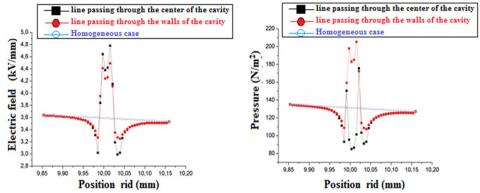Figure 11. Variation of the electric field     Figure 12. Variation of the pressure

Position (rid) = 10 (mm) - Size of the cavity (rc) = 30 (μm)

The size of erosion (re) = 6 (μm)

## 13-14.jpg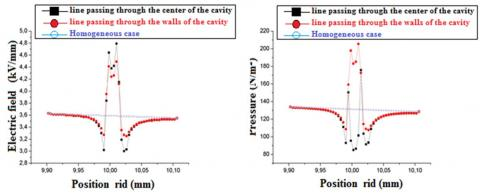Figure 13. Variation of the electric field     Figure 14. Variation of the pressure

Position (rid) = 10 (mm) - Size of the cavity (rc) = 20 (μm)

The size of erosion (re) = 4 (μm)

## 15-16.jpgFigure 15. Variation of the electric field     Figure 16. Variation of the pressure

Position (rid) = 10 (mm), Size of the cavity (rc) = 30  (μm)

## 17-18.jpgFigure 17. Variation of the electric field     Figure 18. Variation of the pressure

Position (rid) = 10 (mm) - Size of the cavity (rc) = 40 (μm)

The size of erosion (re) = 8 (μm)

## 19-20.jpg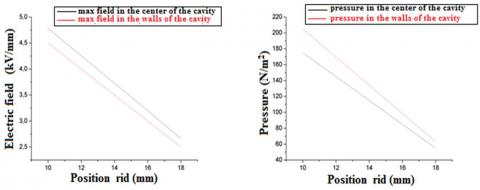Figure 19. Variation of the electric field     Figure 20. Variation of the pressure

depending on the size of the cavity.     depending on the size of the cavity.

Positions (rid) = 10 – 16 – 14 – 18 (mm) - Size of the cavity (rc) = 30 (μm)

The size of erosion (re) = 6 (μm)

## 21-22.jpg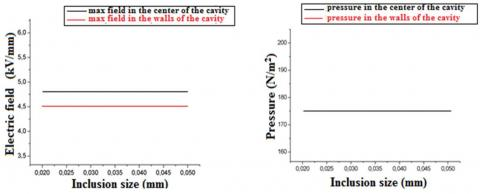Figure 21. Variation of the electric field     Figure 22. Variation of the pressure

depending on the size of the erosion      depending on the size of the erosion

Size of (rc) = 20 – 30 – 40 - 50 (μm) -Position ( rid ) = 10 (μm)

## 23-24.jpgFigure 23. Variation of the electric field     Figure 24.Variation of the pressure

depending on the size of the erosion     depending on the size of the erosion

Position (rid) = 10 (mm) - Size of the cavity (rc) = 30 (μm)

The size of erosion (re) = 6 (μm)

## 25-26.jpgFigure 25. Variation of the electric field     Figure 26. Variation of the pressure

depending on the size of the erosion     depending on the size of the erosion

Position (rid) = 10 (mm) - Size of the cavity (rc) = 30 (μm)

The size of erosion (re)  = 12 (μm)

## 27-28.jpgFigure 27. Variation of the electric field     Figure 28. Variation of the pressure

Position (rid) = 10 (mm) - Size of the cavity (rc) = 30 (μm)

The size of erosion (re) = 18 (μm)

## 29-30.jpgFigure 29. Variation of the electric field     Figure 30. Variation of the pressure

depending on the size of the erosion     depending on the size of the erosion

Position (rid) = 10 (mm) - Size of the cavity (rc) = 30 (μm)

The size of erosion (re) = 0-6-12-18 (μm)

5. The Interpretation of the Result

Figure (1), (3) and (5): we set the cavity size rc = 30 (m m) and the erosion size re = 6 (mm) Figure 5 rid = 10 (mm). For Figures (7), (9) and (11) it is found that the electric field is maximum inside the cavity is decreases slightly sue the walls.  For Figures (8), (10) and (12) the electrostatic pressure is important on the walls of the cavity, however inside the walls of the erosion the field and the electrostatic pressure take values lower than values of these stresses in a homogeneous insulation when the size of the erosion is (1/5) of the size of the cavity. For Figures (13), (14), (15), (16), (17), (18), (19) and (20) for a given position of (10mm) it is found that the size of the cavity has no influence on the values   of the field and the electrostatic pressure within the selected cavity diameter limit (20, 30 and 40 (mm)). For Figures (25) and (26) the electric field and the electrostatic pressure decrease each time one moves away from the cable core. For Figures (21) and (22) it is shown that the size of the cavity has no influence on the values of the field and the electrostatic pressure within the chosen diameter of the cavities (20 and 30 (mm). For Figures (25) and (27) we conclude a high concentration of electric fields inside the erosion as soon as it takes a size (2/5) of the size of the cavity. For Figures (26) and (28) the electrostatic pressure reaches a very large value and concentrated on the walls of erosion in the direction of the electric field. For Figures (29) and (30) it is found that the field and the electrostatic pressure are constant up to the size of 6 (mm) more than this value there is a significant increase to the size of the erosion reaches 12 (mm), (2/5) of the cavity, then it takes a constant value up to the size of 18 (mm). For Figures (21) and (22) it is shown that the size of the cavity has no influence on the values of the field and the electrostatic pressure within the chosen diameter of the cavities (20 and 30 (mm).  For Figures (25) and (27) we conclude a high concentration of electric fields inside the erosion as soon as it takes a size (2/5) of the size of the cavity. For Figures (26) and (28) the electrostatic pressure reaches a very large value and concentrated on the walls of erosion in the direction of the electric field. For Figures (29) and (30) it is found that the field and the electrostatic pressure are constant up to the size of 6 (mm) more than this value there is a significant increase to the size of the erosion reaches 12 (mm), (2/5) of the cavity, then it takes a constant value up to the size of 18 (mm). The relative permittivity of the gas εd = 1, the relative permittivity of the insulator εi.

6. Conclusion

We will summarize our work to study the phenomena that appear most important (partial discharges, erosions, electric tree). After a description on the High voltage cable, and after the representation of the fundamental notions of electrostatics, we began to expose the two methods for calculating the potential and the electric field by numerical methods, among these methods our choice focused on the finite difference method that is best adopted in the study of our case. Despite its drawbacks of approximations such as the restriction of the field of study due to the high number of mesh nodes and the number of iterations that affect the accuracy of the results, this method nevertheless remains an effective tool for the calculation of the potential and the electric field. After having determined the different equations of the electric field in the insulator, in the defect and on the borders of the defect, we elaborated a program in turbo-pascal which allowed us to calculate the variation of the electric field in a cable. At first, we calculated the theoretical values   of the field in the perfect case we calculated the potential by the numerical method, and we checked the validity of our program by comparing the results get to those calculate theoretically. For the case of the defects, we have noted the influence of this defect on the field and the electrostatic pressure and the deformation provoked with respect to the homogeneous case, the results of calculations obtained:

- The electric field and the electrostatic pressure decrease each time one moves away from the cable core, the cavity size has no influence on the values of the two constraints for a given position, and within the diameter of the cavity, the electric field is maximum inside the cavity is decreasing slightly on the walls, the electrostatic pressure is important on the walls of the cavity, On the walls of erosion the field and the electrostatic pressure take values lower than the values of these stresses in the homogeneous insulation. For a given position, note that the cavity size has no influence on the values of the fields and the electrostatic pressure within the limits of the diameters of the cavity chosen.

- There is a high concentration of electric fields inside the erosion as soon as it takes a given size of the cavity size. The repeated action of partial discharges inside the cavity erodes the walls thereof in the direction of the electric field. We chose cavity sizes that can be the partial discharge seat under electrical cable service constraints, indeed work on this subject showed that partial discharges occur for cavities sizes of twenty micro meters whatever the position taken in the insulating envelope. So we took cavities greater than twenty micrometers.

References

 Nouar, (1999). Study of the influence of gaseous cavities and the distribution of the field Electromechanical and electromechanical pressure and dielectric loss in the insulation of cables of high voltage. these magister, The National Polytechnic School.

 Kouidri, M.A., Mahi, D. (1992). Study and realization of an electrostatic precipitator device. Modelling, Measurement and Control C, 79(4): 235-241. https://doi.org/10.18280/mmc_c.790415

 Giam, H.T., Malek, D. (1992). Phenomenon of dielectric breakdown of insulating materials in electrotechnique. 3rd day Magrebine of materials science 96 101, Algiers.

 Beroual, A. (1991). Course of dielectric and high voltage materials. 1st year post graduation, ENP.

 Sergeant, A. (1978). Contribution to the study of solid insulation aging subjected to landfills partial Doctoral thesis 3rd cycle. United Parcel Service, Toulouse.

 Wolzak, G., Van de Laar, A.M.F.J., Steennis, E.F. (1986). Partial discharges and the electrical aging of XLPE cable insulation. research reports, Eindhoven University of Technology, E-160: 1-22.

 Danikas, M.G. Karafyllis, I., Thanailakis, A., Brunuig, A.M. (1996). Simulation of electrical tree growth in solid dielectrics containing voids of arbitrary shape. Modelling and Simulation in Materials Science and Engineering, 4(6): 535-552. https://doi.org/10.1088/0965-0393/4/6/001

 Agoris, D.P., Hatziargyrion, N.D. (1993). Approch to partial discharge development in closely coupled cavities embedded in solid dielectrics by the lumped capacitance model. IEE  Proceedings-A, 140(2): 131-134. https://doi.org/10.1049/ip-a-3.1993.0021

 Guchton, G.C., Karlson, P.W., Pederson, A. (1989). Partial discharges in ellipsoidal and spheroidal voids. IEEE Transaction on electrical insulation, 24(2): 335-342. https://doi.org/10.1109/14.90292

 Bass, J. (1961). Math course. Masson edition 1961.

 Allister, D.M., Smith, J.R., Diserens, M.J. (1994). Computer modelling in electrostatics. edition Research studies press.

 Sibony, M., Mordon, J.C.I. (1988). Numerical analysis - Approximation and differential equations. Tome II, Hermann edition.

 Pelletier, J.P. (1982). Numerical techniques applied to scientific computing. Masson edition.

 Toya, A., Sudo, Y., Motoi, K., Hirotsu, K., Fukunaya, S. (1997). Partial discharges in various voids in XLPE. 10th international symposium on high voltage engineering, Montreal.

 Vlad, S., Mihailescu, M., Rafiroiu, D. (1997). Nummerical field computation for various electrode configurations in plare type electrostatic separators. 10th International Symposium on High Voltage Engineering, Montréal.

 Guibadj, M., Nouar, A., Lefkaier, I.K., Boubakeur, A. (2000). Study by simulation of the phenomenon of the appearance of partial discharges in the insulation of high voltage cables. 1st national seminar in electrical engineering, 74-77.